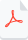# Uniqueness of  Analytic Continuation

The uniqueness property of analytic continuation is a key concept in complex analysis. It asserts that given an analytic function defined on a domain D, there can be only one possible analytic continuation of that function to a larger domain containing D. In other words, any two analytic continuations of a function must agree on their common domain of definition.

This property is closely related to the fact that analytic functions are "well-behaved" in the complex plane. They have a smooth and regular behavior, and any discontinuities or singularities must be isolated points or poles. This allows us to extend the domain of an analytic function in a unique and well-defined way, by "gluing" together local analytic functions defined on overlapping regions.

In the context of the Riemann zeta function and the super symmetric equation, the uniqueness property of analytic continuation ensures that if two different values have been mapped to the same value, it violates the analytic continuation property and, therefore, cannot be accurate.

The uniqueness property of analytic continuation is a result of the fact that analytic functions are uniquely determined by their power series expansions. That is, if f(z) is an analytic function defined in some open set U, and if g(z) is another analytic function defined in a larger open set V that contains U, such that f(z) = g(z) for all z in U, then g(z) is uniquely determined by its Taylor series expansion about any point in U.

To see why this is the case, let's consider two power series expansions:

f(z) = ∑ a_n (z-z_0)^n g(z) = ∑ b_n (z-z_0)^n

where z_0 is some fixed point in U. Since f(z) and g(z) agree on U, it follows that their coefficients must be the same up to some point N:

a_n = b_n for all n ≤ N.

Now, consider the function h(z) = f(z) - g(z). By construction, h(z) vanishes on U, so h(z) has a zero of some order m > 0 at z_0. This means that the first m derivatives of h(z) at z_0 are zero:

h^(k)(z_0) = 0 for all k ≤ m-1.

However, the power series expansions of f(z) and g(z) imply that

h^(k)(z) = a_k - b_k for all k ≥ 0.

Therefore, we have

a_k - b_k = 0 for all k ≤ m-1.

But this means that the Taylor series expansions of f(z) and g(z) must agree up to order m-1:

f(z) = g(z) + O((z-z_0)^m).

Since this holds for any z in U, it follows that the Taylor series expansions of f(z) and g(z) must be identical. Therefore, the function g(z) is uniquely determined by its power series expansion, and we say that it is the analytic continuation of f(z) to the larger domain V.

ζ(s) = ζ(1-s*)

Suppose that there exist two analytic functions f(z) and g(z) that both satisfy this equation in some open set U. By the uniqueness property of analytic continuation, we know that f(z) and g(z) must agree on any larger open set V that contains U. Therefore, the function f(z) = g(z) is uniquely determined by its power series expansion about any point in U. But this means that ζ(s) has a unique analytic continuation to any larger domain that contains U, and therefore the zeros of ζ(s) are uniquely determined by their power series expansions. This is the basis for the Riemann Hypothesis and its connection to the super symmetric equation.

https://proofwiki.org/wiki/Uniqueness_of_Analytic_Continuation

## Zeros of Analytic Function are Isolated

"Zeros of Analytic Function are Isolated" means that if an analytic function f(z) has a zero at a point z0, then there exists a small neighborhood around z0 where f(z) has no zeros other than z0 itself. In other words, the zeros of an analytic function are isolated points. This property is important in complex analysis, and is used in the study of the distribution of zeros of functions such as the Riemann zeta function.

Theorem: Let f(z) be an analytic function on an open set U. If z0 is a zero of f(z), then there exists a neighborhood of z0 in which f(z) has no zeros other than z0.

Proof: Let z0 be a zero of f(z), which means that f(z0) = 0. Suppose for the sake of contradiction that there exist other zeros of f(z) in a neighborhood of z0. Then we can write f(z) = (z - z0)g(z) where g(z) is analytic and nonzero in a neighborhood of z0.

Since g(z) is nonzero in a neighborhood of z0, it follows that g(z) has a reciprocal, 1/g(z), which is also analytic in that neighborhood. Thus, we can write f(z) = (z - z0)/[1/g(z)].

Now consider the limit of f(z) as z approaches z0. We have:

lim(z→z0) f(z) = lim(z→z0) (z - z0)/[1/g(z)] = (z0 - z0)/[1/g(z0)] = 0/∞ = 0

This shows that f(z) approaches 0 as z approaches z0. But since f(z) is analytic, it follows that f(z) must be identically 0 in some open disk centered at z0. This contradicts the assumption that there exist other zeros of f(z) in a neighborhood of z0, and hence the theorem is proved.

Reference: Ahlfors, L. V. (1979). Complex Analysis: An Introduction to the Theory of Analytic Functions of One Complex Variable. New York: McGraw-Hill.Uniqueness_of_Analytic_Continuation.pdf